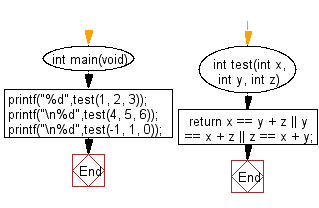﻿ C exercises: Check whether it is possible to add two integers to get the third integer from three given integers - w3resource# C Exercises: Check whether it is possible to add two integers to get the third integer from three given integers

## C-programming basic algorithm: Exercise-23 with Solution

Write a C program to check whether it is possible to add two integers to get the third integer from three given integers.

C Code:

``````#include <stdio.h>
#include <stdlib.h>
int main(void){
printf("%d",test(1, 2, 3));
printf("\n%d",test(4, 5, 6));
printf("\n%d",test(-1, 1, 0));
}
int test(int x, int y, int z)
{
return x == y + z || y == x + z || z == x + y;
}
``````

Sample Output:

```1
0
1
```

Pictorial Presentation:Flowchart:C Programming Code Editor: